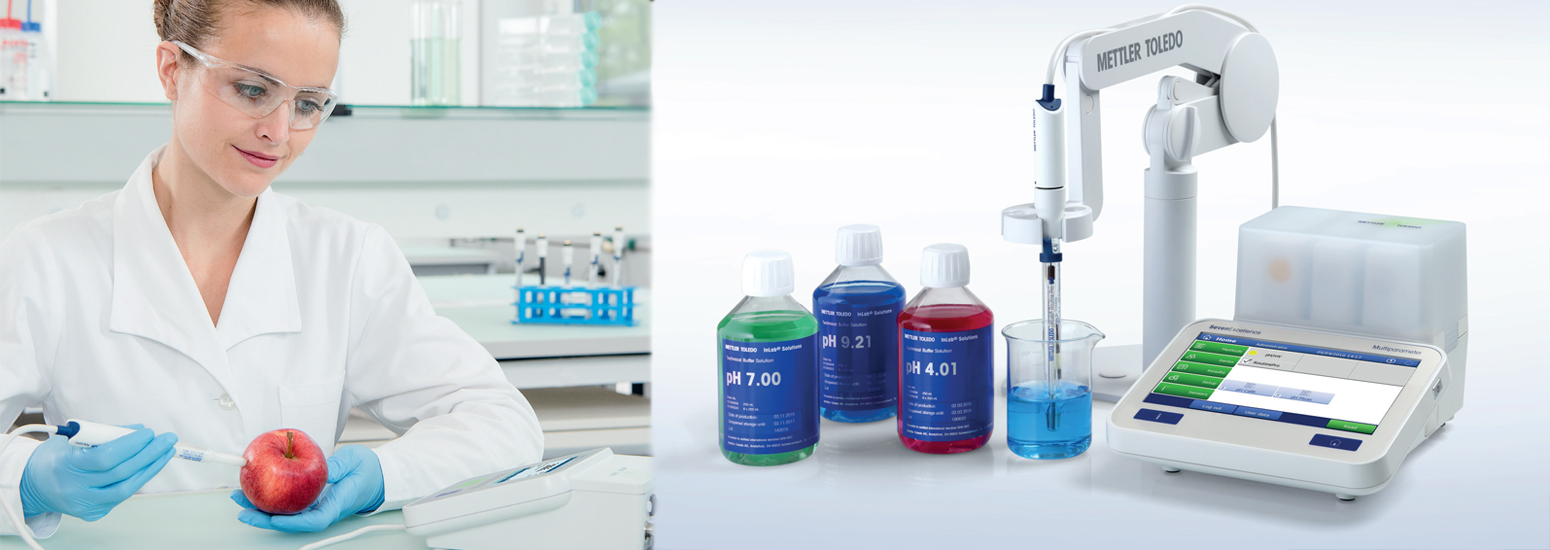# pH Measurement Theory vol1

Introduction to pH

Why do we classify an everyday liquid like vinegar as being acidic? The reason for this is that vinegar contains an excess of hydronium ions (H3O+) and this excess of hydronium ions in a solution makes it acidic. An excess of hydroxyl ions (OH) on the other hand makes something basic or alkaline. In pure water the hydroniumn ions are all neutralized by hydroxyl ions and this solution is what we call at a neutral pH value.

H3O+ OH ↔ 2 H2O

Figure 1.

The reaction of an acid and a base forms water. If the molecules of a substance release hydrogen ions or protons through dissociation we call this substance an acid and the solution becomes acidic. Some of the most well-known acids are hydrochloric acid, sulfuric acid and acetic acid or vinegar. The dissociation of vinegar is shown below:

CH3COOH + H2O ↔ CH3COO + H3O+

Figure 2. Dissociation of acetic acid.

Not every acid is equally strong. Exactly how acidic something is, is determined by the total number of hydrogen ions in the solution. The pH value is then defined as the negative logarithm of the hydrogen ion concentration. (To be precise, it is determined by the activity of the hydrogen ions. See chapter 4.2 for more information on the activity of hydrogen ions).

pH = –log [H3O+]

Figure 3. The formula for calculating the pH value from the concentration of hydronium ions.

The quantitative difference between acidic and alkaline substances can be determined by performing pH value measurements.